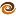# EmptyCrate

#### About Me | Contact Me | Training |ChaiScript.com | YouTube Channel

This is the first in a series where I am going to read through the O’Reilly C++ In A Nutshell language reference and blog about each part. This is mainly for me, to make sure I fully understand the language, and for you, if you care.

This first one will be like a few others where I will group a set of pieces into one entry. For instance, this is all of the operators instead of today blogging about ‘and’ and tomorrow blogging about ‘or’. Although, I will call out the cast operators, throw and new/delete into a separate posting

Operators

• `and`

This is the logical and operator, equivalent to `&&`. And returns true if both sides evaluate to true.

• `and_eq`

equivalent to `&=` and should more appropriately be named “bitand_eq”. `A &= B` is equivalent to `A = A & B`. However, they are two different operators and are overloaded separately, so it is possible for them to have two different meanings.

• `bitand`

`bitand`, which is the same as `&`, performs a bitwise and of the two operands. For instance `0x02 & 0x01 == 0x00`, because 2 and 1 share no bits in common. Also, `0x02 & 0x03 == 0x02` because the ‘2’ bit is shared.

• `bitor`

bitor, equivalent to `|`, performs a bitwise or of the two operands. So, `0x02 | 0x03 == 0x03`. If a bit is in either operand, it is in the result.

• `compl`

compl, equivalent to `~`, performs a bitwise complement of the operand. For instance, `~0x01 == 0xFE` (the result has every bit set EXCEPT for the bits in the operand).

• `not`

not, equivalent to `!`, performs a logical negation. `!true == false`. It is of note that any non 0 value in C++ resolves to true. Therefor `!5 == false` `!0 == true`.

• `not_eq`

not_eq is equivalent to `!=`. `true != false`, `5 != 4` both evaluate to `true`. `not_eq` is a different operator from `!(5 == 4)` and could be overloaded separately in a class.

• `or`

or, equivalent to `||`, returns `true` if either operand evalutes to `true`. For instance `(true || false) == true`, `(false || false) == false`.

• `or_eq`

or_eq, which should be named bitor_eq, is equivalent to `|=` and performs the same operation as `A = A | B`. Note, like the other *_eq operators, it is technically different from the above example.

• `sizeof`

sizeof returns the number of bytes needed to store an object. For instance `sizeof(char) == 5`, `sizeof(int) = 4` (on most 32bit platforms). It is of note that the size may be larger than you are expecting. Some platforms require padding of data between members of a struct, for instance. Also, classes containing virtual functions are larger than classes not containing virtual functions. Thirdly, it should be representative of the actual data being stored. That is, adding a new non-virtual function should not increase the sizeof(classname), because the function’s code is stored at the class level, not the object.

• `typeid`

Returns a const reference to a type_info object which contains information about the object or type referenced.

• `xor`

xor, equivalent to `^`, performs a bitwise exclusive or on the two operands. That is, a bit is only set in the result if it is one operand or the other, but not both. So, `0x02 ^ 0x01 == 0x03`. `0x03 ^ 0x01 == 0x02`.

• `xor_eq`

Equivalant to `^=` which is almost equivalent to `A = A ^ B`. See other _eq operators above.

My Videos

Related